# AP Chemistry : pH

## Example Questions

### Example Question #11 : P H

A chemist boils off the water from 627 mL of a solution with a pH of 10.2. The only dissolved compound in the solution is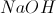. How much solid remains?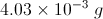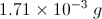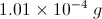No solid remains.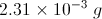Explanation:

The pH of the solution is 10.2, and we know: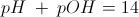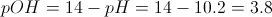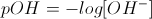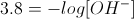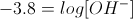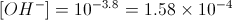And since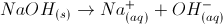,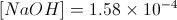.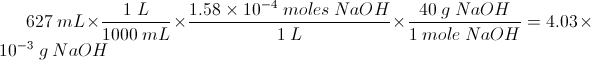### Example Question #11 : P H

A chemist adds 10mL of a solution with a pH of 3.00 to 50mL of water. What is the pH of the new solution?

4.25

3.52

2.22

3.78

3.012

3.78

Explanation:

First, we will determine the amount of hydronium ions in the first solution. This allows us to measure the moles of hydronium ion that are transferred to water.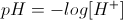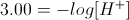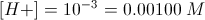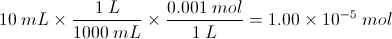Now we can calculate the hydronium ion concentration in the new solution, using the amount transferred and the volume of water, and can solve for pH.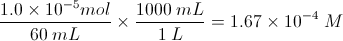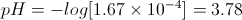### Example Question #11 : P H

Which two solutions, when combined in equal amounts, will produce the lowest pH?

2.0M HCl and 2.0M KOH

1.0M HCl and 1.0M NaCl

2.5M NH3 and 2.5M NH4+

0.5M acetic acid and 1.0M NaCl

1.0M HCl and 1.0M NaCl

Explanation:

HCl is a strong acid, while NaCl is a salt. Mixing these two will result in a pH < 1.

As for the other choices, mixing 2.0M HCl and 2.0M KOH will result in the formation of KCl and H2O, resulting in a neutral pH. Acetic acid (pKa= 4.76) is a weak acid, so it will have a pH less than 7 but greater than 1. Finally, NH3 and NH4+ is a buffer system between a weak base and weak acid, and if equal amounts are present, the pH will be the same as the pKa of NH4+, which is around 9.

### Example Question #11 : Reactions And Equilibrium

1mol of hydrobromic acid (HBr) is added to water, resulting in 1L of solution. Worried that the acid is too strong, a professor adds more water, increasing the volume of solution to 2L.

What was the pH of the solution before adding water? What was the pH after adding the water?

Before, pH = 0; after, pH = 0.3

Before, pH = 1; after, pH = 2

Before, pH = 0; after, pH =-0.3

Before, pH = 1; after, pH = 0.5

Before, pH = 0; after, pH = 0.3

Explanation:

The pH of a solution is given by the equation.

1) We can assume that hydrobromic acid, as a strong acid, will dissociate completely. As a result, there will be 1mol of protons in the initial solution. Molarity is a method of measuring the concentration of an agent in a solution and is defined by the equation below.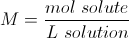Since we have 1mol of protons and 1L of solution, the molarity of protons is simply 1M in the initial solution (1mol/1L). We can plug this into the pH equation and solve.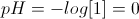2) After the dilution with water, the concentration of protons will change. Since the volume of the solution has doubled, the concentration of protons has been halved.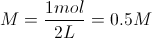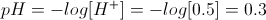* Key points to remember!

1) The lower the pH, the more acidic the solution. This is why the diluted solution was 0.3 and the original, more acidic solution was 0.

2) pH is a logarithmic scale. As a result, a pH of 2 is ten times more acidic than a pH of 3. This is why halving the concentration DID NOT halve the pH.

### Example Question #11 : P H

What is the pH of a solution if you dilute 10mL of a 0.56M NaOH solution into 100mL of water?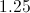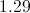There is not enough information to answer this question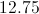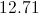Explanation:

We first need to calculate the moles of NaOH in 10mL of a 0.56M solution.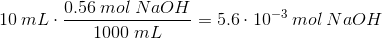Now, in the new volume, the concentration is equal to this same number of moles divided by the new volume. The new volume is the sum of the two solutes together, so the sum of 10mL of solution and 100mL of water.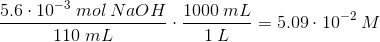Now we need to calculate pH. Since NaOH will dissociate one hydroxide ion for every molecule of NaOH in solution, the concentration of hydroxide is equal to the concentration of NaOH.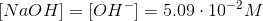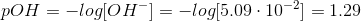Knowing the pH allows us to solve for the pH.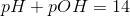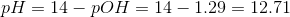### Example Question #12 : P H

What is the pOH of a solution that has a [H+] = 3.2 X 10-7 mol?

3.2

6.5

14

7.5

-7

7.5

Explanation:

[H+] = 3.2 X 10-7 mol

pH = -log [H+]

= 7 - log 3.2

= 7 -.505

=6.495

pOH = 14 - pH

= 7.5

### Example Question #12 : P H

What is the range of possible hydrogen ion concentrations in basic solution?

10–13 to 10–7.001

7

7 to 14

2 to 3

10–1 to 10–6.999

10–13 to 10–7.001

Explanation:

For a solution to be basic, it must have a pH between 8 and 14, since 7 is neutral; pH is –log(hydrogen ion concentration); the range of possible values is between 10–13 and 10–7.001.

### Example Question #13 : Acid Base Reactions

A solution has a hydrogen ion concentration of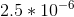. What is the pH of this solution?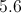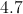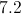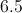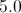Explanation:

pH can be found by taking the negative log of the hydrogen ion concentration.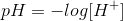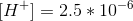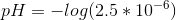We may not know the exact value of this calculation, but we know that it will be somewhere between 5 and 6.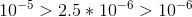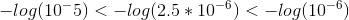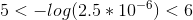### Example Question #14 : Acid Base Reactions

Find the pH of a 0.03M solution of nitric acid.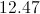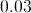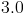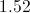Explanation:

Nitric acid is a strong acid, meaning that it will dissociate completely in water.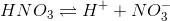As a result, a 0.03M nitric acid solution will dissociate completely, resulting in a proton concentration of 0.03M.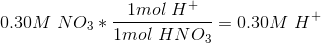Now that we know the proton concentration, we can use the equation for pH to find the pH value of the solution.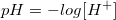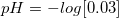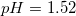### Example Question #15 : Acid Base Reactions

Find the pH of a 0.1M solution of hydrogen cyanide.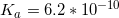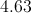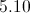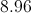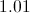Explanation:

Hydrogen cyanide will dissociate according to the following reaction.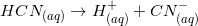Hydrogen cyanide is a weak acid, meaning that it will not dissociate completely in solution. We need to use the acid dissociation constant in order to determine the concentration of protons in solution at equilibrium. We can solve for this value using an ICE table.

I. The initial concentration for the acid of 0.1M. There are no protons or cyanide ions in the solution initially, so they both have concentrations of 0.0M.

C. The concentration of both ions will increase by an unknown amount,, while the acid concentration will decrease by a concentration of.

E. Write the equilibrium expression and compare it to the dissociation constant.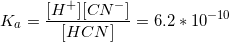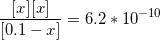The value ofwill be small enough that it is negligible in comparison to 0.1, and can be ignored in the denominator.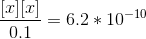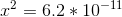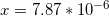From our set up, we know that the value ofis equivalent to the concentration of hydrogen ions at equilibrium. Using this value, we can calculate the pH of the solution.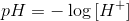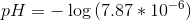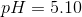### All AP Chemistry Resources